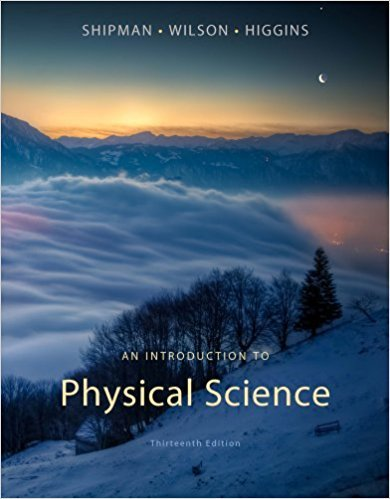×
×

# Which is a major source of air pollution? (20.4)(a)ISBN: 9781133109099 229

## Solution for problem 10 Chapter 20

An Introduction to Physical Science | 13th Edition

• Textbook Solutions
• 2901 Step-by-step solutions solved by professors and subject experts
• Get 24/7 help from StudySoup virtual teaching assistantsAn Introduction to Physical Science | 13th Edition

4 5 1 385 Reviews
21
2
Problem 10

Which is a major source of air pollution? (20.4)(a) nuclear electrical generation(b) incomplete combustion(c) temperature inversion(d) acid rain

Step-by-Step Solution:
Step 1 of 3

Class 6 – Vectors in Physics Dr. Ilia Gogoladze Physics by Walker, 4 edition Adding Vectors - Two ways to add vectors o Graphically  Tip to tail or head to tail  The resultant is drawn from the origin of the first vector to the end of the last vector  When you have many vectors,...

Step 2 of 3

Step 3 of 3

##### ISBN: 9781133109099

The answer to “Which is a major source of air pollution? (20.4)(a) nuclear electrical generation(b) incomplete combustion(c) temperature inversion(d) acid rain” is broken down into a number of easy to follow steps, and 18 words. This textbook survival guide was created for the textbook: An Introduction to Physical Science, edition: 13. An Introduction to Physical Science was written by and is associated to the ISBN: 9781133109099. This full solution covers the following key subjects: . This expansive textbook survival guide covers 24 chapters, and 736 solutions. Since the solution to 10 from 20 chapter was answered, more than 223 students have viewed the full step-by-step answer. The full step-by-step solution to problem: 10 from chapter: 20 was answered by , our top Physics solution expert on 01/09/18, 07:57PM.

Unlock Textbook Solution